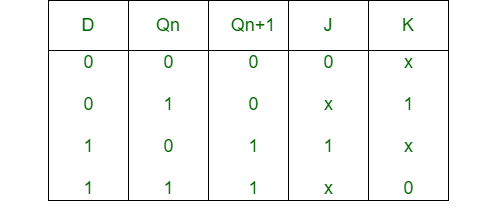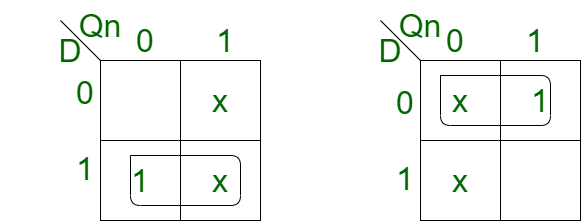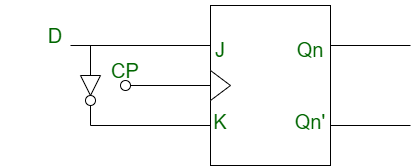# Conversion of J-K Flip-Flop into D Flip-Flop

• Difficulty Level : Hard
• Last Updated : 22 Apr, 2020

Prerequisite – Flip-flop

1. JK Flip-Flop:
JK Flip-Flip is basically a gated SR flip-flop which has an additional input that is clock input. It prevents the invalid output that may be obtained when both the inputs are 1.

2. D Flip-Flop:
D Flip-Flop is a modified SR flip-flop which has an additional inverter. It prevents the inputs from becoming the same value.

Conversion of J-K Flip-Flop into D Flip-Flop:

• Step-1:
We construct the characteristic table of D flip-flop and excitation table of JK flip-flop.• Step-2:
Using the K-map we find the boolean expression of J and K in terms of D.```J = D
K = D' ```
• Step-3:
We construct the circuit diagram of the conversion of JK flip-flop into D flip-flop.My Personal Notes arrow_drop_up